Normal view MARC view ISBD view

# Contemporary abstract algebra / Joseph A. Gallian, University of Minnesota Duluth.

Material type:BookCopyright date: Boston, MA : Cengage Learning, 2017Edition: Ninth edition.Description: xvi, 557, 43 pages : illustrations ; 24 cm.ISBN: 9781305657960 (hardback); 1305657969 (hardback).DDC classification: 512.02
Partial contents:
Part 1. Integers and equivalence relations -- Preliminaries -- Part 2. Groups -- 1. Introduction to groups -- 2. Groups -- 3. Finite groups; subgroups -- 4. Cyclic groups -- 5. Permutation groups -- 6. Isomorphisms -- 7. Cosets and Lagrange's Theorem -- 8. External direct products -- 9. Normal subgroups and factor groups -- 10. Group homomorphisms -- 11. Fundamental theorem of finite Abelian groups -- Part 3. Rings -- 12. Introduction to rings -- 13. Integral domains -- 14. Ideals and factor rings -- 15. Ring homomorphisms -- 16. Polynomial rings -- 17. Factorization of polynomials -- 18. Divisibility in integral domains -- Part 4. Fields -- 19. Vector spaces -- 20. Extension fields -- 21. Algebraic extensions -- 22. Finite fields -- 23. Geometric constructions -- Part 5. Special topics -- 24. Sylow theorems -- 25. Finite simple groups -- 26. Generators and relations -- 27. Symmetry groups -- 28. Frieze groups and crystallographic groups -- 29. Symmetry and counting -- 30. Cayley digraphs of groups -- 31. Introduction to algebraic coding theory -- 32. An introduction to Galois Theory -- 33. Cyclotomic extensions.
Abstract: his book is primarily intended for an abstract algebra course whose main purpose is to enable students to do computations and write proofs. The text stresses the importance of obtaining a solid introduction to the traditional topics of abstract algebra, while at the same time presenting it as a contemporary and very much an active subject which is currently being used by working physicists, chemists, and computer scientists.
Tags from this library: No tags from this library for this title.
Item type Current location Call number Copy number Status Date due Item holdsLibro académico
512.02 G168c 2017 (Browse shelf) Ej. 1 Available
Total holds: 0
##### Browsing Biblioteca del Campus Shelves Close shelf browser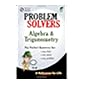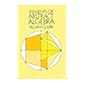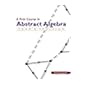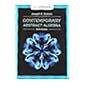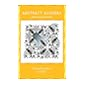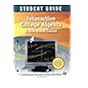512.0076 A394 2000 The algebra & trigonometry problem solver : 512.02 C5921e 1984 Elements of abstract algebra / 512.02 F812f 2003 A first course in abstract algebra / 512.02 G168c 2017 Contemporary abstract algebra / 512.02 H5729a 1999 Abstract algebra / 512.02 J932a 2016 Abstract algebra : 512.0711 F529i 2005 Interactive college algebra :

Includes indexes.

Includes bibliographical references.

Part 1. Integers and equivalence relations -- Preliminaries -- Part 2. Groups -- 1. Introduction to groups -- 2. Groups -- 3. Finite groups; subgroups -- 4. Cyclic groups -- 5. Permutation groups -- 6. Isomorphisms -- 7. Cosets and Lagrange's Theorem -- 8. External direct products -- 9. Normal subgroups and factor groups -- 10. Group homomorphisms -- 11. Fundamental theorem of finite Abelian groups -- Part 3. Rings -- 12. Introduction to rings -- 13. Integral domains -- 14. Ideals and factor rings -- 15. Ring homomorphisms -- 16. Polynomial rings -- 17. Factorization of polynomials -- 18. Divisibility in integral domains -- Part 4. Fields -- 19. Vector spaces -- 20. Extension fields -- 21. Algebraic extensions -- 22. Finite fields -- 23. Geometric constructions -- Part 5. Special topics -- 24. Sylow theorems -- 25. Finite simple groups -- 26. Generators and relations -- 27. Symmetry groups -- 28. Frieze groups and crystallographic groups -- 29. Symmetry and counting -- 30. Cayley digraphs of groups -- 31. Introduction to algebraic coding theory -- 32. An introduction to Galois Theory -- 33. Cyclotomic extensions.

his book is primarily intended for an abstract algebra course whose main purpose is to enable students to do computations and write proofs. The text stresses the importance of obtaining a solid introduction to the traditional topics of abstract algebra, while at the same time presenting it as a contemporary and very much an active subject which is currently being used by working physicists, chemists, and computer scientists.

There are no comments for this item.